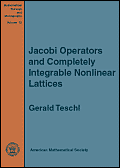Book
Mathematical Surveys and Monographs, Volume 72, Amer. Math. Soc., Providence 2000.

## Jacobi Operators and Completely Integrable Nonlinear Lattices

### Gerald Teschl

Abstract
This book is intended to serve both as an introduction and a reference to spectral and inverse spectral theory of Jacobi operators (i.e., second order symmetric difference operators) and applications of these theories to the Toda and Kac-van Moerbeke hierarchy.

Starting from second order difference equations we move on to self-adjoint operators and develop discrete Weyl-Titchmarsh-Kodaira theory, covering all classical aspects like Weyl m-functions, spectral functions, the moment problem, inverse spectral theory, and uniqueness results. Next, we investigate some more advanced topics like locating the essential, absolutely continuous, and discrete spectrum, oscillation theory, trace formulas, random operators, almost periodic operators, (quasi-)periodic operators, scattering theory, and spectral deformations.

Then, the Lax approach is used to introduce the Toda hierarchy and its modified counterpart, the Kac-van Moerbeke hierarchy. Uniqueness and existence theorems for the initial value problem, solutions in terms of Riemann theta functions, the inverse scattering transform, Bäcklund transformations, and soliton solutions are discussed.

Three appendices review the theory of compact Riemann surfaces, Herglotz functions, and show how one can use a program for symbolic computation, Mathematica, to do some of the work (Jacobi equations with Mathematica).

MSC91: 39Axx, 47B39, 37Kxx
Keywords: Jacobi operators, Toda hierarchy, Kac-van Moerbeke hierarchy.

Ordering DetailsPublisher: American Mathematical Society Series: Mathematical Surveys and Monographs, ISSN: 0076-5376 Volume: 72 Publication Year: 2000 ISBN: 0-8218-1940-2 / 978-0-8218-1940-1 Paging: 351 pp; hardcover List Price: \$68 Member Price: \$54 Itemcode: SURV/72
Order from AMS Book Store
Errata
An errata containing known mistakes is available. If you also found a mistake, or if you have any comments, please mail them to me!
The AMS has granted the permisson to make the full book available as dvi file (2.4M) or pdf file (1.9M).

Permission is granted to retrieve, print, and store a single copy for personal use only. If you like this book and want to support the idea of online versions, please consider buying this book. Note that all known mistakes are corrected in the online version, other than that it is identical to the printed version.

Preface

#### Part 1: Jacobi Operators

1. Jacobi operators
1. General properties
2. Jacobi operators
3. A simple example
4. General second order difference expressions
5. The infinite harmonic crystal in one dimension
2. Foundations of spectral theory
1. Weyl m-functions
2. Properties of solutions
3. Positive solutions
4. Weyl circles
5. Canonical forms of Jacobi operators and the moment problem
6. Some remarks on unbounded operators
7. Inverse spectral theory
3. Qualitative theory of spectra
1. Locating the spectrum and spectral multiplicities
2. Locating the essential spectrum
3. Locating the absolutely continuous spectrum
4. A bound on the number of eigenvalues
4. Oscillation theory
1. Prüfer variables and Sturm's theorem
2. Classical Oscillation theory
3. Renormalized oscillation theory
5. Random Jacobi operator
1. Random Jacobi operators
2. The Lyaponov exponent and the density of states
3. Almost periodic Jacobi operators
6. Trace formulas
1. Asymptotic expansions
2. General trace formulas and xi functions
7. Periodic Jacobi operators
1. Floquet theory
2. Connections with the spectra of finite Jacobi operators
3. Polynomial identities
4. A simple example - continued
5. Perturbations of periodic operators
8. Reflectionless Jacobi operators
1. Spectral analysis and trace formulas
2. Isospectral operators
3. The finite-gap case
4. Further spectral interpretation
9. Quasi-periodic Jacobi operators
1. Riemann surfaces
2. Solutions in terms of theta functions
3. The elliptic case, genus one
4. Illustrations of the Riemann-Roch theorem
10. Scattering theory
1. Transformation operators
2. The scattering matrix
3. The Gel'fand-Levitan-Marchenko equations
4. Inverse scattering theory
11. Spectral deformations
1. Commuting first order difference expressions
2. The single commutation method
3. Iteration of the single commutation method
4. Application of the single commutation method
5. A formal second commutation
6. The double commutation method
7. Iteration of the double commutation method
8. The Dirichlet deformation method
12. Notes on literature

#### Part 2: Completely Integrable Nonlinear Lattices

13. The Toda system
1. The Toda lattice
2. Lax pairs, the Toda hierarchy, and hyperelliptic curves
3. Stationary solutions
4. Time evolution of associated quantities
14. The initial value problem
1. Finite-gap solutions of the Toda hierarchy
2. Quasi-periodic finite-gap solutions and the time-dependent Baker-Akhiezer function
3. A simple example - continued
4. Inverse scattering transform
5. Some additions in case of the Toda lattice
6. The elliptic case - continued
15. The Kac-van Moerbeke system
1. The Kac-van Moerbeke hierarchy and its relation to the Toda hierarchy
2. Kac and van Moerbeke's original equations
3. Spectral theory for supersymmetric Dirac-type difference operators
4. Associated solutions
5. N-soliton solutions on an arbitrary background
16. Notes on literature

#### Appendix

1. Compact Riemann surfaces - a review
1. Basic notation
2. Abelian differentials
3. Divisors and the Riemann-Roch theorem
4. Jacobian variety and Abel's map
5. Riemann's theta function
6. The zeros of the Riemann theta function
7. Hyperelliptic Riemann surfaces
2. Herglotz functions
3. Jacobi equations with Mathematica
1. The package DiffEqs and first order difference equations
2. The package JacDEqs and Jacobi difference equation
3. Simple properties of Jacobi difference equations
4. Orthogonal Polynomials
5. Recursions
6. Commutation methods
7. Toda lattice
8. Kac-van Moerbeke lattice
4. Bibliography
Glossary of notations
Index